# Printable Number Line TemplateUpdated on Sep 28, 2022By Printablee Team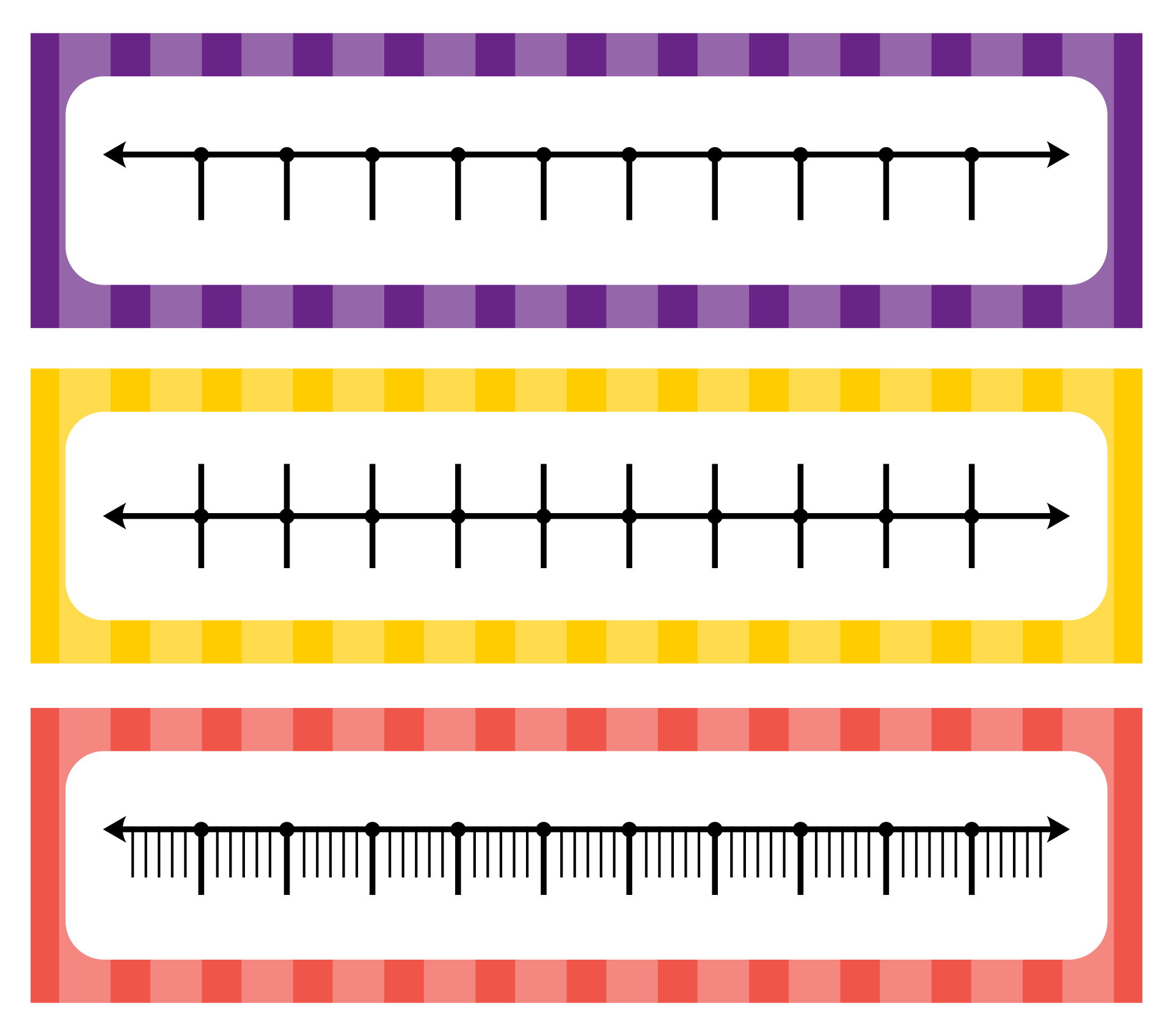### How do you arrange a number line worksheet?

To make number learning more fun, you can go with the number line. By learning the number line, kids will understand how to use the ruler system and other things that can measure height and weight on a number scale.

Using the worksheet in applying the number line is such a great idea. If you need ideas for constructing your own worksheet, just go straight on and get inspired!

Arranging the kids to trace the number in the number line worksheet is such a great idea. After you introduce the kids to the number line, it’s better to remind them but still make them play part in it.

Let the kids trace each number you provide in the worksheet. Fill-in-the-blank worksheet could be a great task for reminding them of the number line. This worksheet makes the kids fill the blank space with the appropriate number they find through the number clues.

After the kids already stick with the number line template, you can arrange the activity that makes them create the whole number line.

Before jumping to give the task, better to teach the kids in using the ruler first. For arranging this task, all you need to prepare is the blank worksheet with the instruction and also the number range. Let the kids use the ruler to create the line and also the sign for placing the number.

Those are how you can arrange the number worksheet. Make sure the kids already understand the concept of numbers before jumping to this task.

### How can you use addition and subtraction in the number line worksheet?

To make the kids stick with math learning, you can start with numbering first. There are various ways you can introduce the kids to it.

Using the number line is one of the options. If the kids are already familiar with numbering, you can involve the math formulas as the task the kids need to work with. If you want to know further, let’s dive right in!

Let’s go with the addition first. you will need the number line template and the additional questions. Using the grid with two columns will be so much help. The grid can consist of the additional question on the left and the number line on the right.

Instruct the kids to do the addition first. after they have the result, kids have to give the sign on the number line. For example, there will be the addition of 5 + 5. Because the result is 10, kids have to give the circle sign on the 10 number.

For the subtraction, basically, it’s all the same but the kids have to find the result of the subtraction first and give the circle sign in the number line.

Before you jump to construct the worksheet, make sure you already teach the kids about the number line system. Give them an explanation that the kids only can look at the number line to have the result of addition and subtraction.

For the addition, they can just go with the first number on the question and count as the amount of the second number in the right direction. Meanwhile, for the subtraction, they have to go in the left direction.

That’s how you can arrange the addition and subtraction number line worksheet. Make sure the kids already attach to the number line system so they can execute it without any doubt.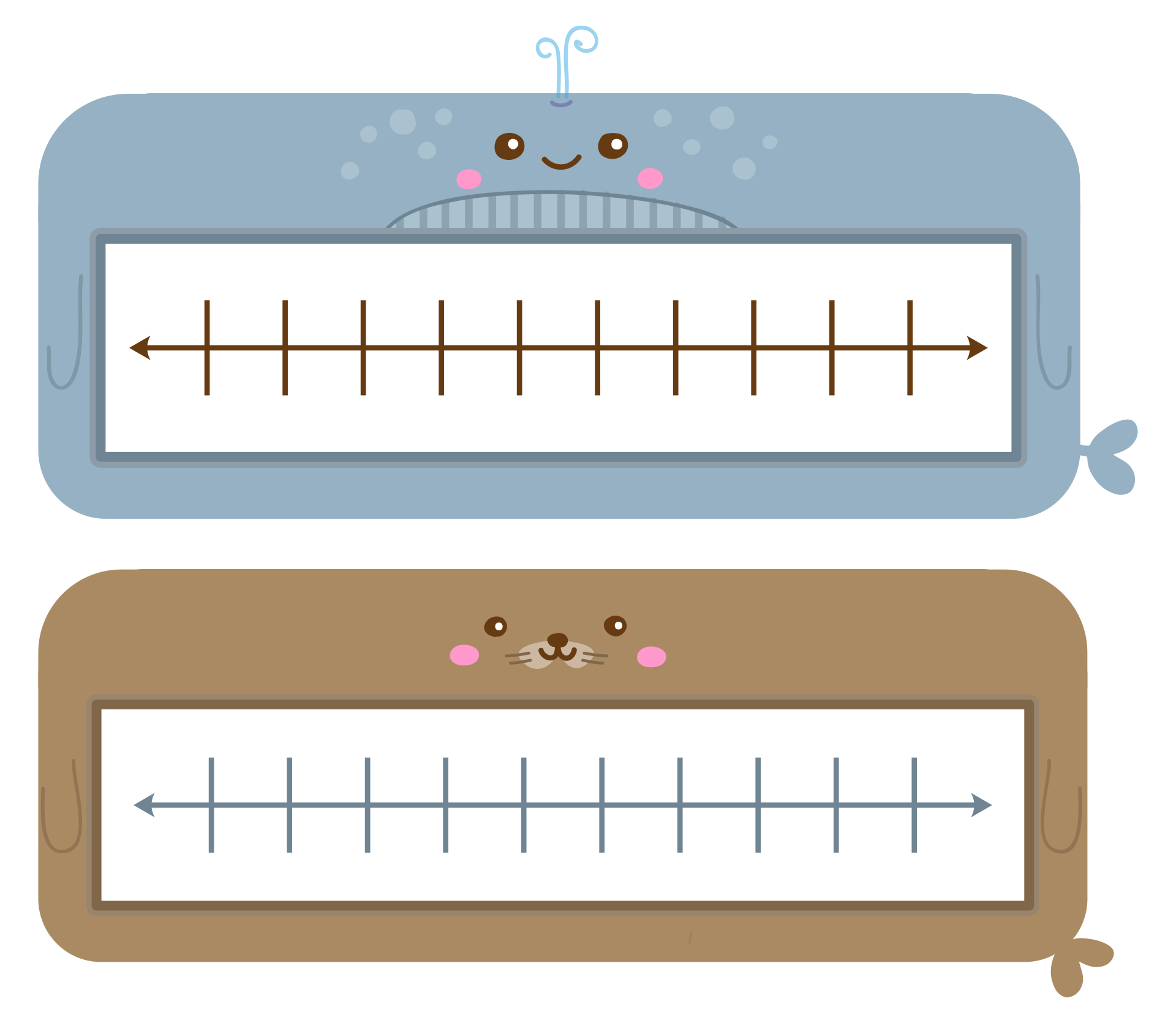We also have more printable number you may like:
Printable Number Line 1-100
Hundreds Number Line Printable
Printable Number Line 0 50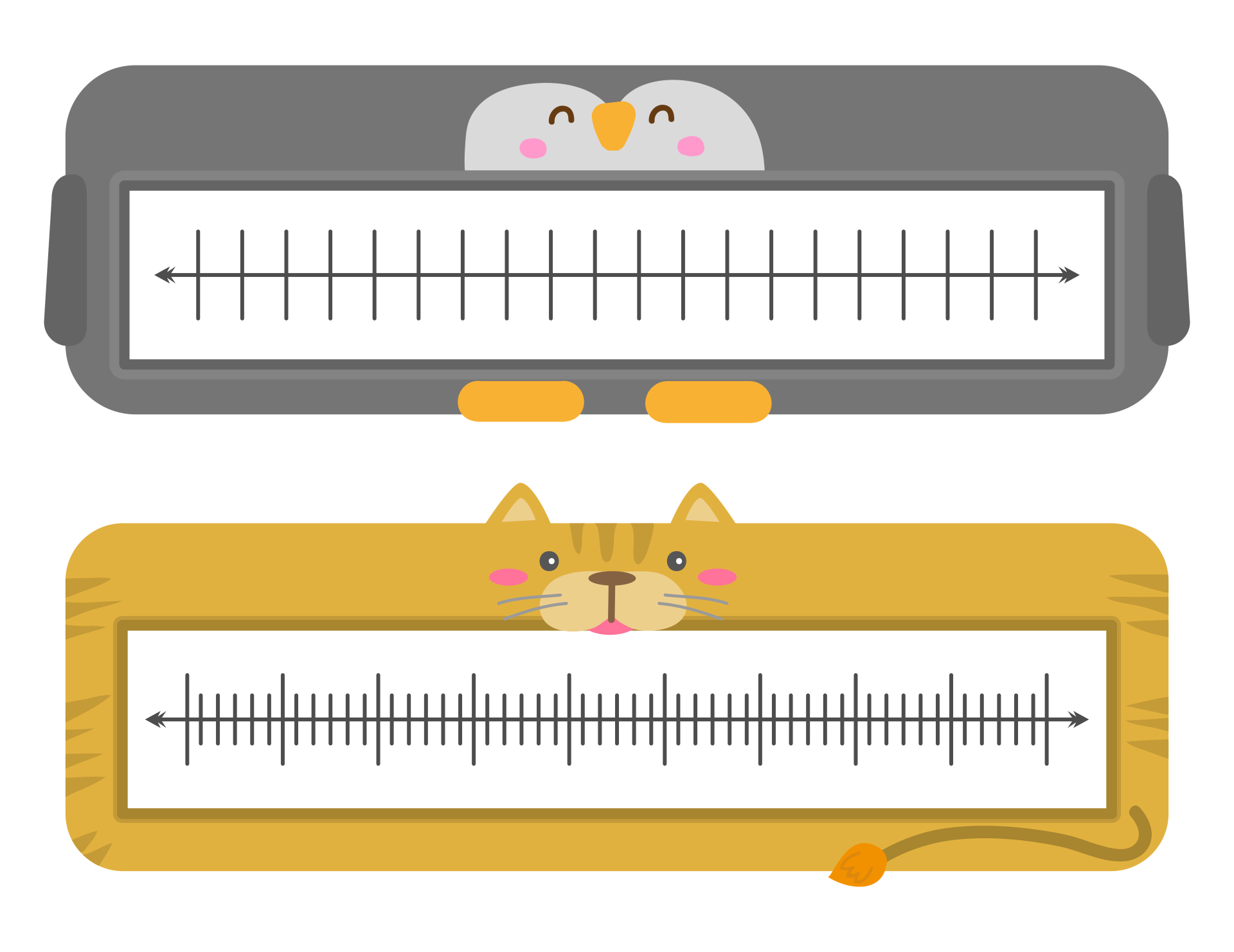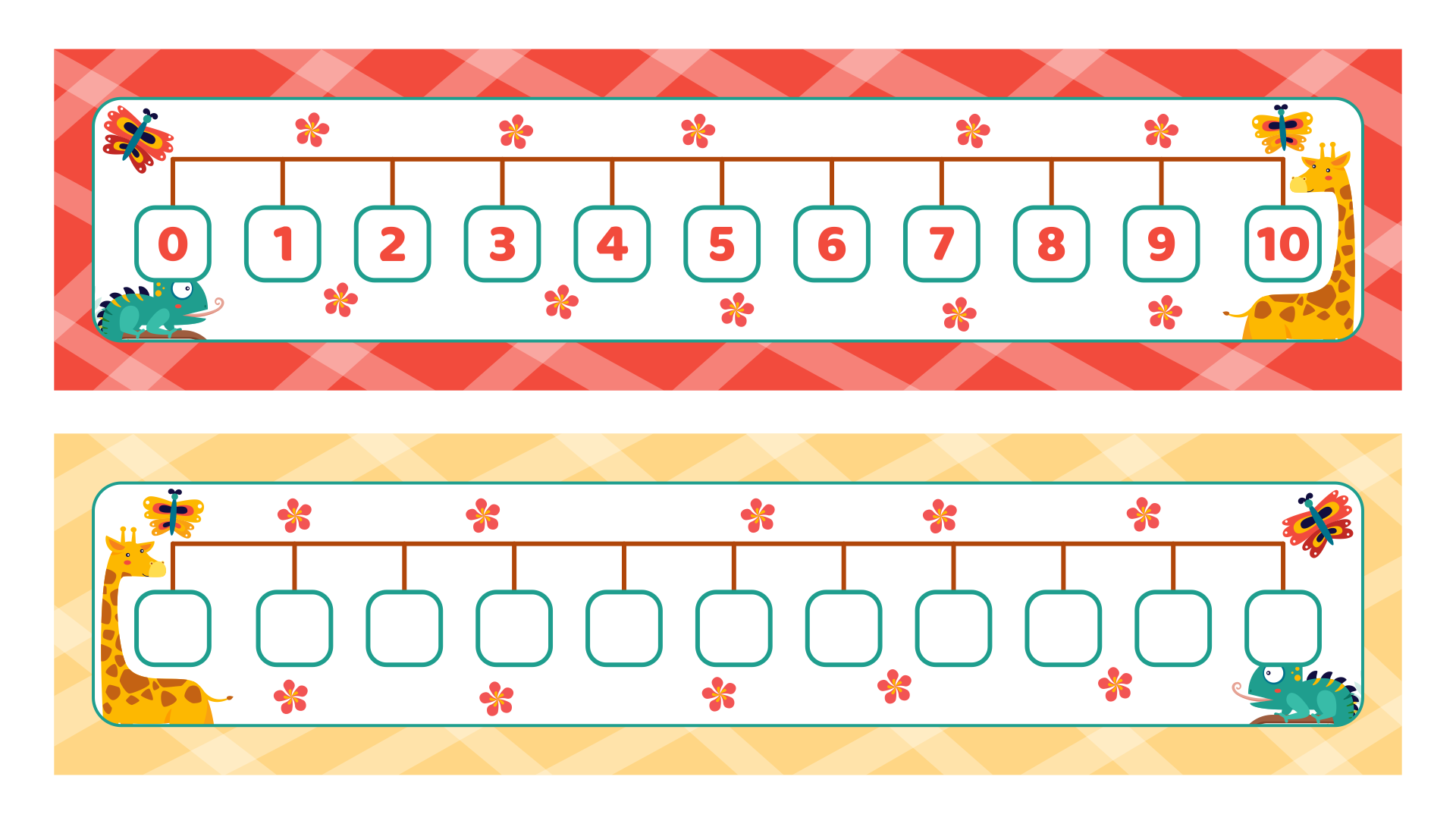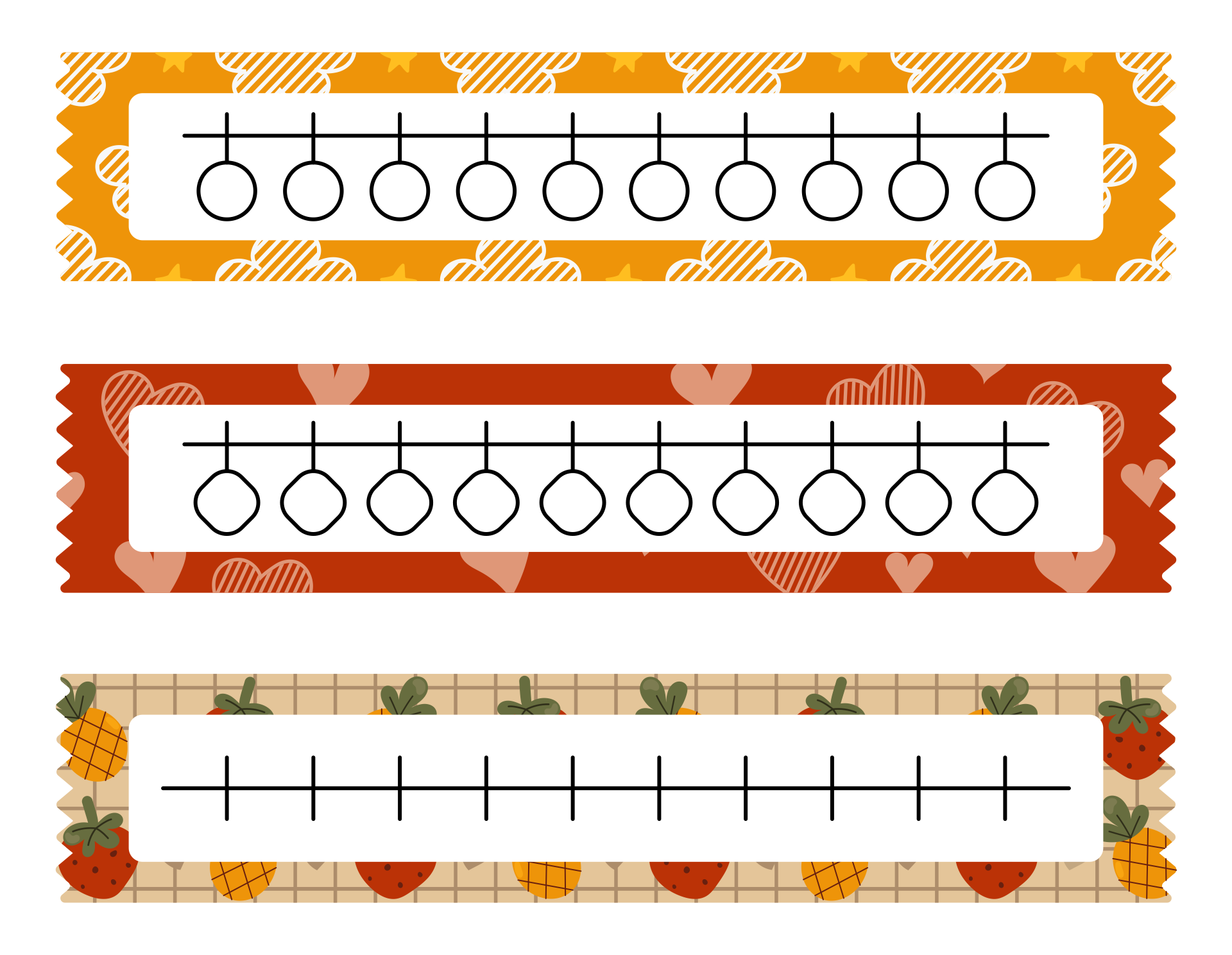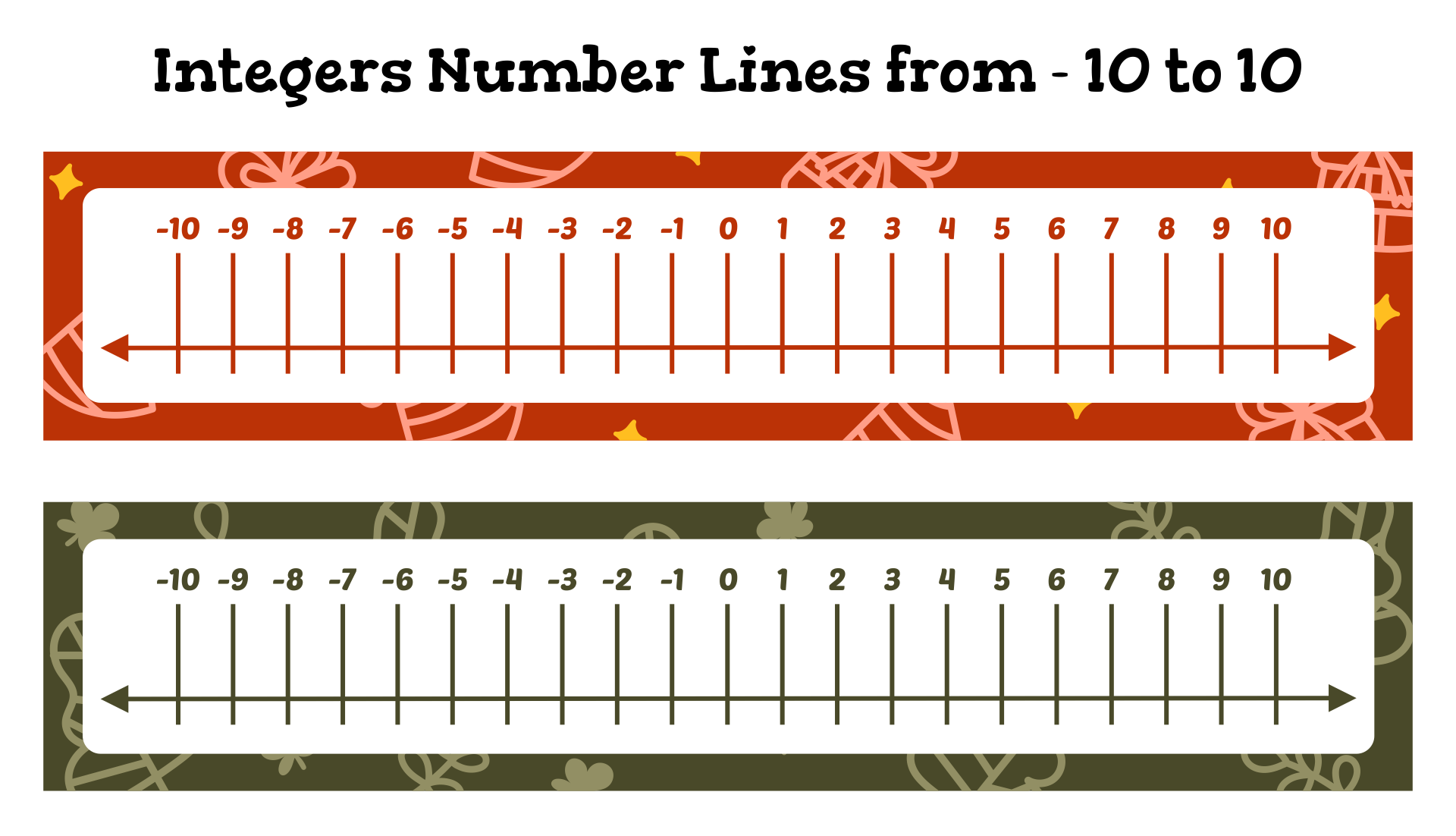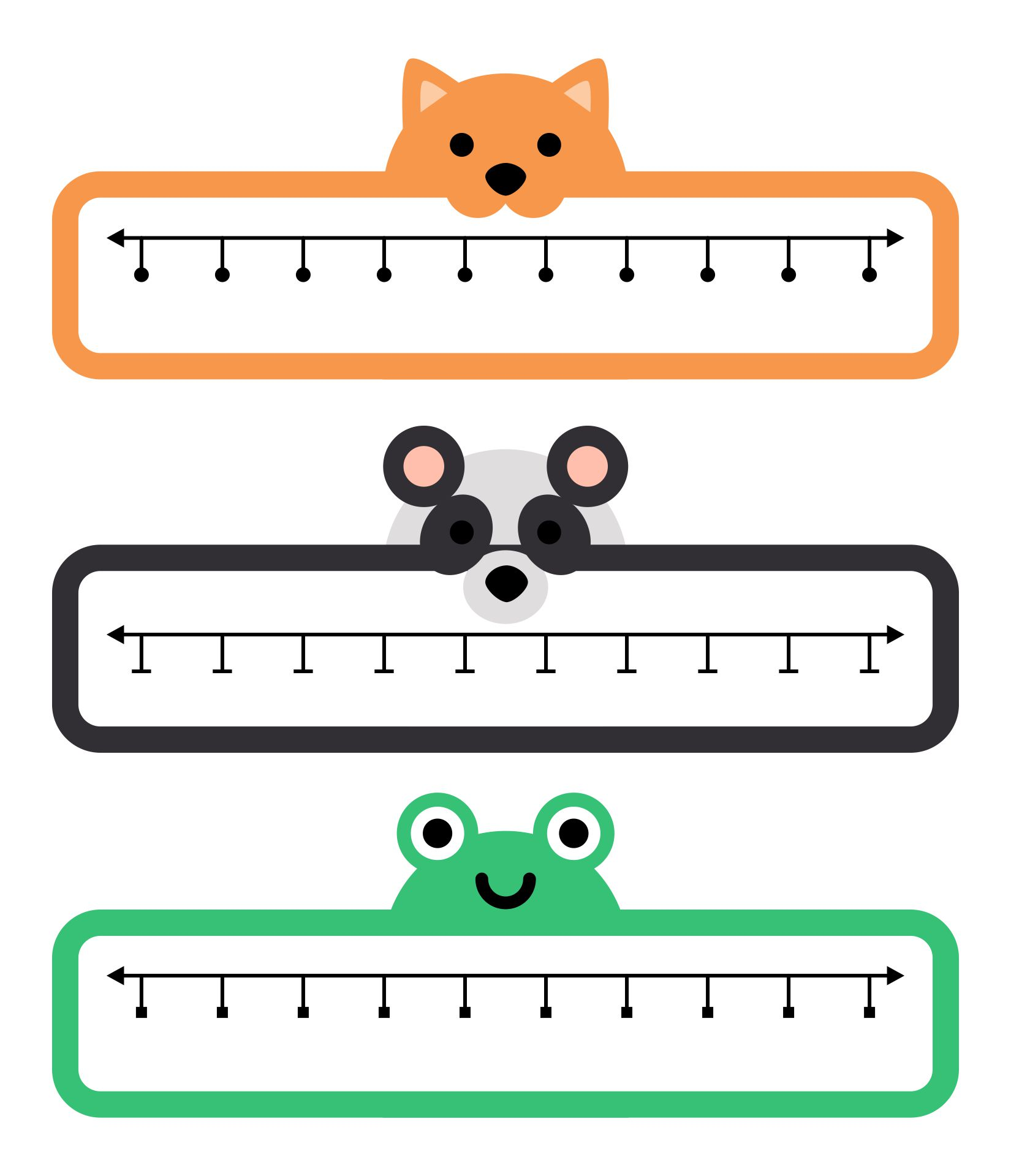### What can I include in the number sense worksheet?

The role of the worksheet is important in any aspect of learning. By using the worksheet, you can directly test the material you’ve tach with this tool.

It’s in line with number learning. You can arrange for the kids to have a great numbering sense through its worksheet. Let’s dive right in to know the activities inside!

The number sense worksheet consists of some activities related to numbers that kids have to do. The first activity can be making the kids define the number. Provide the table with the category of hundreds, tens, and ones.

All the kids need to do is place each number question on the table as it should. Continue with the coloring activity.

Have the grids for the kids’ color as the amount of the number. Basically, the kids have to count and color each grid so it will be in the right amount as the number.

Tracing task is great to include topo. You can arrange for the kids to arrange the number along with the number words in it. So, you will provide the dot to dot of number and the number words on the worksheet. Have a task for the kids to write the number after and before.

For example, you are having the task of number 15. So, the kids need to find the number before which is 14, and the number after which is 16.

The number line task is appropriate to include too. you can let the kids give the circle sign in the number of the task question.

Those are what you can involve in the number sense worksheet. If you consider constructing the number sense worksheet, attach those with you and elaborate on your knowledge.